Aptitude Tests 4 Me

Download Free EBooks for Various Types of Aptitude Tests

Non Verbal Logic

Detailed solution

111.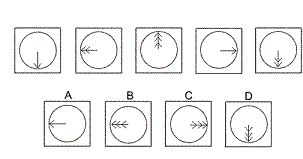Solution: B Explanation: In this question, there are two rules to follow. The first rule is that the arrow rotates by 90° clockwise each time. Following this rule, the next diagram of the sequence should have the arrow pointing towards 9 o’clock. Therefore, the correct answer could be A or B. The other rule is that the number of arrowheads changes from 1 to 2 to 3, then back to 1 again etc. Following this rule, the number of arrowheads in the next diagram should be three. Therefore, the correct answer is B.

112.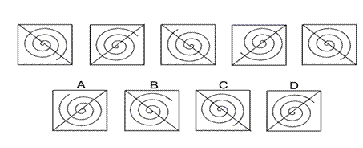Solution: D Explanation: In this question, there are two rules to follow. The first rule is that the spiral rotates by 90° anticlockwise each time. Following this rule, the next diagram of the sequence should have the tail of the spiral at about 2 o’clock. Therefore, the correct answer could be B or D. The other rule is that the diagonal alternates from to right-bottom left to top rightbottom left. Following this rule, the diagonal in the next diagram should be top right-bottom left. Therefore, the correct answer is D.

113.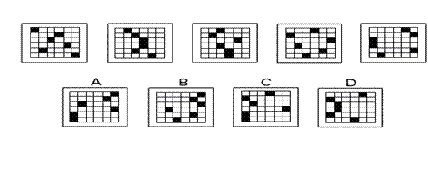Solution: A Explanation: In this question, there is one black square in each row of the grid. The black squares in the first, third and fifth rows move one place to the right each time; and when they reach the right edge of the grid, they move to the first column of the left edge. The black squares in the second, fourth and sixth rows move one place to the left each time; and when they reach the left edge of the grid, they move to the first column of the right edge. Therefore, the correct answer is A.

114.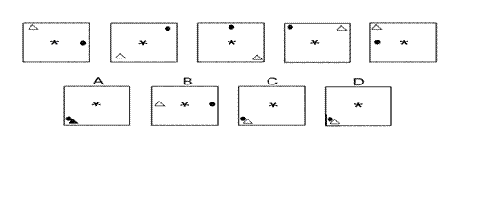Solution: C Explanation: In this question, there are three rules to follow. The first rule is that the asterisk flips vertically each time. Following this rule, in the next diagram of the sequence, the asterisk will be upside down. Therefore, the correct answer could be A, B or C. The second rule concerns the white triangle which rotates each time through 90° anticlockwise around the outside of the square. Following this rule, in the next diagram of the sequence, the white triangle should be in the bottom left corner of the square. Therefore, the correct answer could be C or D The third rule concerns the black dot which rotates each time through 45° anticlockwise around the outside of the square. Following this rule, in the next diagram of the sequence, the black dot should be in the bottom left corner of the square. Therefore, the correct answer could be A, C or D Taking all the rules into consideration, the only possible answer is C.

115.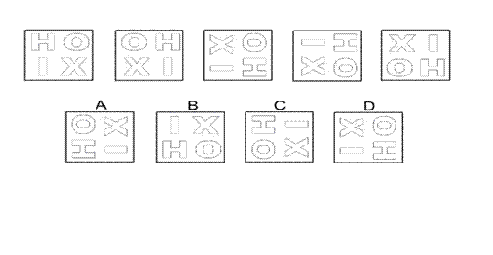Solution: B Explanation: In this question, there is a sequence of transformations: ‘reflection in the vertical axis’, ‘rotation of 90° clockwise’, ‘reflection in the horizontal axis’, ‘rotation of 90° clockwise’, ‘reflection in the vertical axis’ etc Following this sequence, the next transformation should be ‘reflection in the vertical axis’, which means the next diagram in the sequence will be B.

116.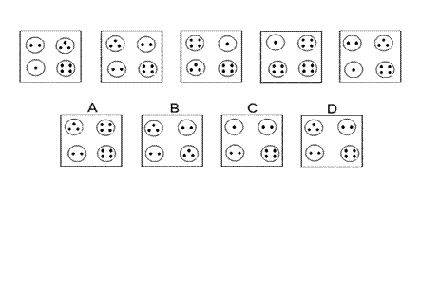Solution: D Explanation: In this question, the number of dots in each of the four circles follows a rule: For the circle in the top left corner, the number of dots increases by 1 each time, with 4 becoming 1. For the circle in the top right corner, the number of dots decreases by 1 each time, with 1 becoming 4. For the circle in the bottom left corner, the number of dots increases by 1 each time, with 4 becoming 1. For the circle in the bottom right, the number of dots is always 4. Following these rules, for the next diagram in the sequence there should be 3 dots in the top left circle, 2 dots in the top right circle, 2 dots in the bottom left circle and 4 dots in the bottom right circle. Therefore, the correct answer is D.

117.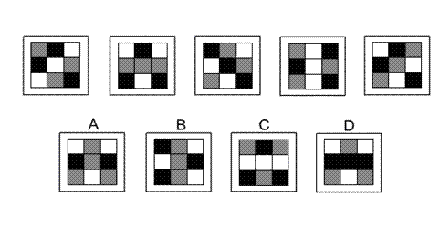Solution: D Explanation: In this question, there are two rules to follow. The first rule is that the colours of the squares follow a pattern: black squares change to white, grey change to black and white change to grey. The second rule is that the eight squares around the perimeter move one place clockwise each time. Both these rules have to be applied simultaneously. Following these rules, the next diagram in the sequence must be D.

118.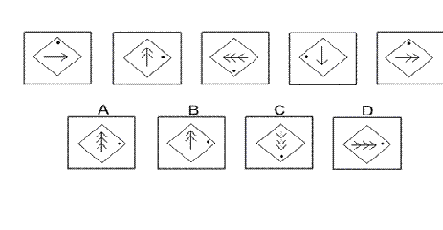Solution: A Explanation: In this question, there are three rules to follow. The first rule is that the dot moves through 90° clockwise each time. Following this rule, in the next diagram of the sequence, the dot should be at 3 o’clock. Therefore, the correct answer could be A, B or D. The second rule is that the line with arrows rotates through 90° anticlockwise each time. Following this rule, in the next diagram of the sequence, the arrowheads should point to 12 o’clock. Therefore, the correct answer could be A or B. The third rule is that the number of arrowheads increases by 1 each time, with three arrowheads becoming 1. Following this rule, in the next diagram of the sequence, there should be three arrowheads. Therefore, the correct answer is A.

119.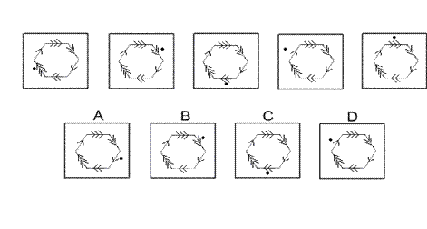Solution: C Explanation: In this question, there is a hexagon with arrowheads on each of its sides. There is also a dot. The dot rotates clockwise around the perimeter of the hexagon according to the number of arrowheads it is adjacent to. Following this rule, to find the next diagram of the sequence, the dot will have to rotate three places around the perimeter of the hexagon. Therefore, the correct answer is C.

120.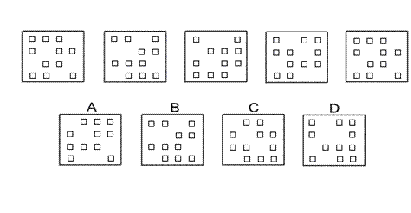Solution: B Explanation: In this question, there is a grid of squares. There are two rules that must be followed, one after the other. The first rule is: the columns of the grid are moved one place to the right with the fourth column moving to the first column. The second rule is: the rows of the grid are moved one place up with the top row moving to the bottom row. Following these rules, to get the next diagram in the sequence will be B. 11.b. A directory is a listing of names or things, so choice b is the essential element. A telephone (choice a) often has a directory associated with it, but it is not essential. A computer (choice c) uses a directory format to list files, but it is Not required.Names (choice d) are often listed in a directory, but many other things are listed in directories, so this is not the essential element.

 1 2 3 4 5 6 7 8 9 10 11 12 13 14 15 16 17 18 19 20 21 22 23 24 25 26 27 28 29 30 31 32 33 34 35 36 37 38 39 40 41 42 43 44 45 46 47 48 49 50 51 52 53 54 55 56 57 58 59 60 61 62 63 64 65 66 67 68 69 70 71 72 73 74 75 76 77 78 79 80 81 82 83 84 85 86 87 88Passage ReadingVerbal LogicNon Verbal LogicNumerical LogicData InterpretationReasoningAnalytical AbilityBasic NumeracyAbout UsContactPrivacy PolicyMajor TestsFAQ